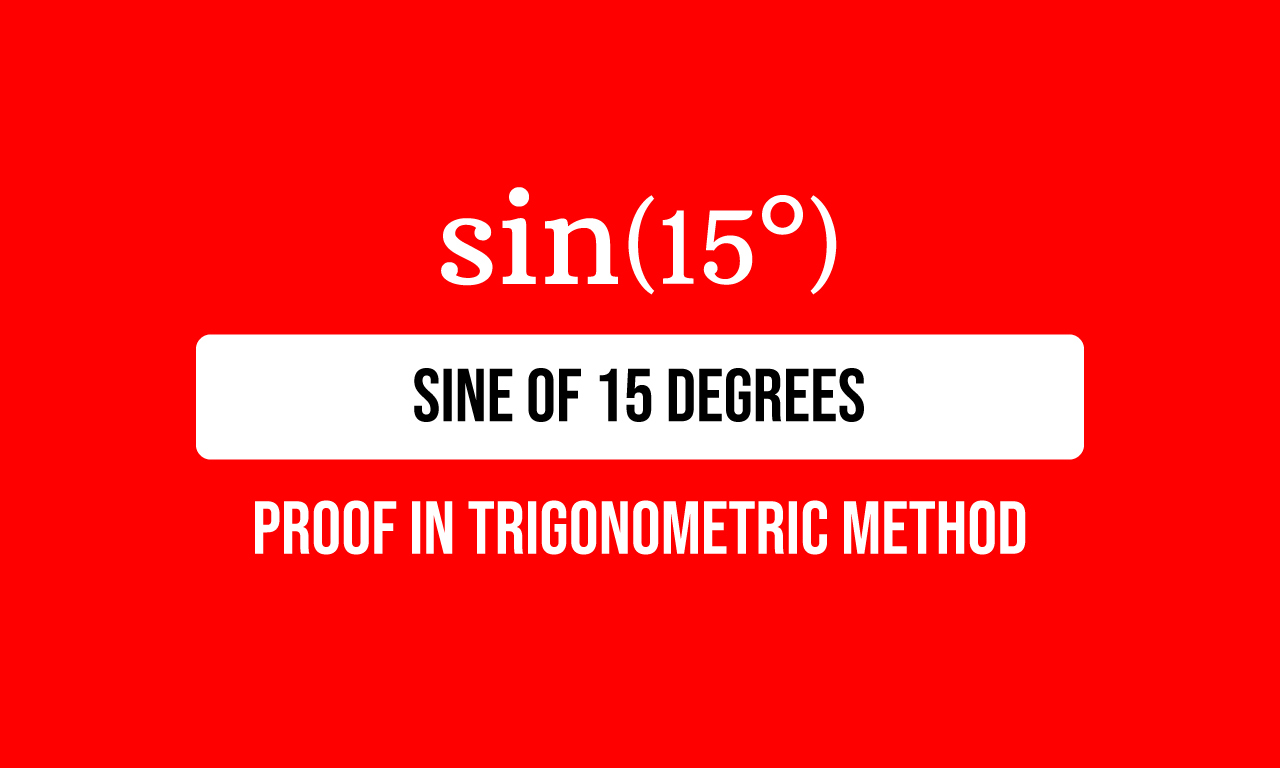# Proof of $\sin{(15^\circ)}$ in Trigonometric method

The sine of fifteen degrees is exactly equal to the square root of three minus one divided by two times square root of two. It is also equal to the square root of six minus square root of two divided by four.$\sin{(15^\circ)}$ $\,=\,$ $\dfrac{\sqrt{3}-1}{2\sqrt{2}}$ $\,=\,$ $\dfrac{\sqrt{6}-\sqrt{2}}{4}$

It is an exact value of sine of angle $15$ degrees in fraction form and It can be derived exactly by a trigonometric identity and the values of trigonometric functions for some standard angles. Now, let’s learn how to derive the exact value of sine $15$ degrees in trigonometry.

### Split the angle as a Compound angle

The angle fifteen degrees can be obtained by subtracting a standard angle thirty degrees from another standard angle forty five degrees. So, sine of angle $15$ degrees can be written as sine of a compound angle $45$ degrees minus $30$ degrees.

$\implies$ $\sin{(15^\circ)}$ $\,=\,$ $\sin{(45^\circ-30^\circ)}$

### Expand the sine of difference of angles

The sine of the difference of two angles forty five degrees and thirty degrees represents the sine of difference of angles. It can be expanded as per the angle difference identity of sine functions.

$\implies$ $\sin{(45^\circ-30^\circ)}$ $\,=\,$ $\sin{45^\circ}\cos{30^\circ}$ $\,-\,$ $\sin{30^\circ}\cos{45^\circ}$

### Simplify the Trigonometric expression

The sine of angle $15$ degrees is expanded as a trigonometric expression in terms of sine and cosine of angles $45$ and $30$ degrees, and this trigonometric expression is used to find the exact value of sine of $15$ degrees by simplification.

$=\,\,\,$ $\sin{45^\circ} \times \cos{30^\circ}$ $\,-\,$ $\sin{30^\circ} \times \cos{45^\circ}$

According to the trigonometry, the values of sine of forty five degrees, cosine of thirty degrees, sine of thirty degrees and cosine of forty five degrees are known to us. So, substitute the value of each trigonometric function in the above trigonometric expression.

$=\,\,\,$ $\dfrac{1}{\sqrt{2}} \times \dfrac{\sqrt{3}}{2}$ $\,-\,$ $\dfrac{1}{2} \times \dfrac{1}{\sqrt{2}}$

Now, multiply the fractions in each term to simplify the quantities in the terms of the expression.

$=\,\,\,$ $\dfrac{1 \times \sqrt{3}}{\sqrt{2} \times 2}$ $\,-\,$ $\dfrac{1 \times 1}{2 \times \sqrt{2}}$

$=\,\,\,$ $\dfrac{1 \times \sqrt{3}}{2 \times \sqrt{2}}$ $\,-\,$ $\dfrac{1 \times 1}{2 \times \sqrt{2}}$

$=\,\,\,$ $\dfrac{\sqrt{3}}{2\sqrt{2}}$ $\,-\,$ $\dfrac{1}{2\sqrt{2}}$

$=\,\,\,$ $\dfrac{\sqrt{3}-1}{2\sqrt{2}}$

$\therefore\,\,\,$ $\sin{(15^\circ)}$ $\,=\,$ $\dfrac{\sqrt{3}-1}{2\sqrt{2}}$

Therefore, the sine of angle fifteen degrees is equal to the square root of three minus one divided by two times square root of two.

$\therefore\,\,\,$ $\sin{(15^\circ)}$ $\,=\,$ $\dfrac{\sqrt{6}-\sqrt{2}}{4}$

Latest Math Topics
Jun 26, 2023
Jun 23, 2023

###### Math Questions

The math problems with solutions to learn how to solve a problem.

Learn solutions

Practice now

###### Math Videos

The math videos tutorials with visual graphics to learn every concept.

Watch now

###### Subscribe us

Get the latest math updates from the Math Doubts by subscribing us.